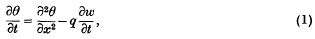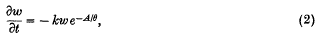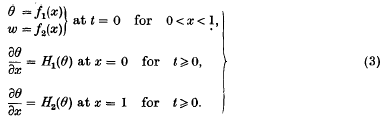Hostname: page-component-cd4964975-8tfrx Total loading time: 0 Render date: 2023-03-30T01:08:11.916Z Has data issue: true Feature Flags: { "useRatesEcommerce": false } hasContentIssue true

# A practical method for numerical evaluation of solutions of partial differential equations of the heat-conduction type

Published online by Cambridge University Press:  24 October 2008

## Extract

This paper is concerned with methods of evaluating numerical solutions of the non-linear partial differential equationwheresubject to the boundary conditionsA, k, q are known constants.

Equation (1) is of the type which arises in problems of heat flow when there is an internal generation of heat within the medium; if the heat is due to a chemical reaction proceeding at each point at a rate depending upon the local temperature, the rate of heat generation is often defined by an equation such as (2).

Type
Research Article
Information

## Access options

Get access to the full version of this content by using one of the access options below. (Log in options will check for institutional or personal access. Content may require purchase if you do not have access.)

## References

#### REFERENCES

(1)Hartree, D. R. and Womersley, J. R.Proc. Roy. Soc. A, 161 (1937), 353.CrossRefGoogle Scholar
(2)Jackson, R., Sarjant, R. J., Wagstaff, J. B., Eyres, N. R., Hartree, D. R. and Ingham, J. The Iron and Steel Institute, 1944, Paper No. 15/1944 of the Alloy Steels Research Committee.Google Scholar
Eyres, N. R., Hartree, D. R. and others. Philos. Trans. A, 240 (1946), 1.CrossRefGoogle Scholar
(3)Richardson, L. F.Philos. Trans. A, 210 (1910), 307.CrossRefGoogle Scholar
(4)Richardson, L. F.Philos. Trans. A, 226 (1927), 299.CrossRefGoogle Scholar
(5) A.M.P. Memo. No. 131. IM: Title ‘On the solution of certain boundary problems’.Google Scholar
(6)Levy, and Baggott, . Numerical Studies in Differential Equations, Chapter iv.Google Scholar
(7)Lowan, A. N.Amer. J. Math. 56, no. 3 (1934), 396.CrossRefGoogle Scholar
(8)Bamford, C. H., Crank, J. and Malan, D. H.Proc. Cambridge Phil. Soc. 42 (1946), 166.CrossRefGoogle Scholar